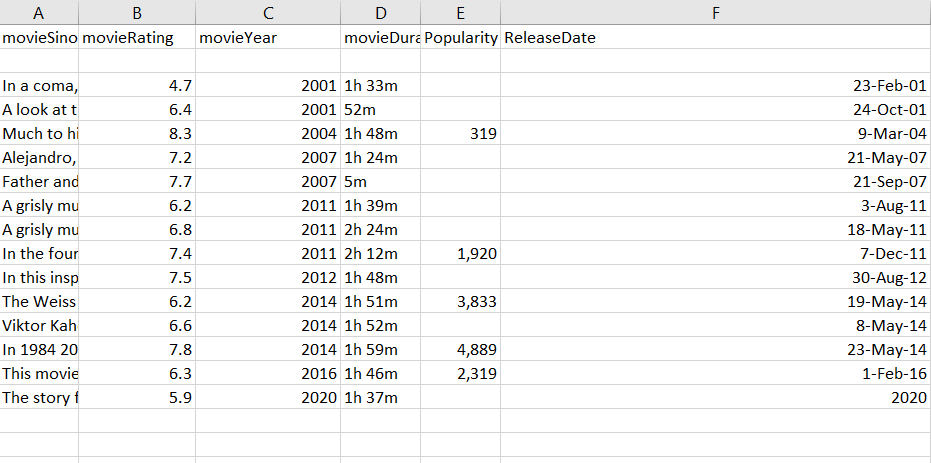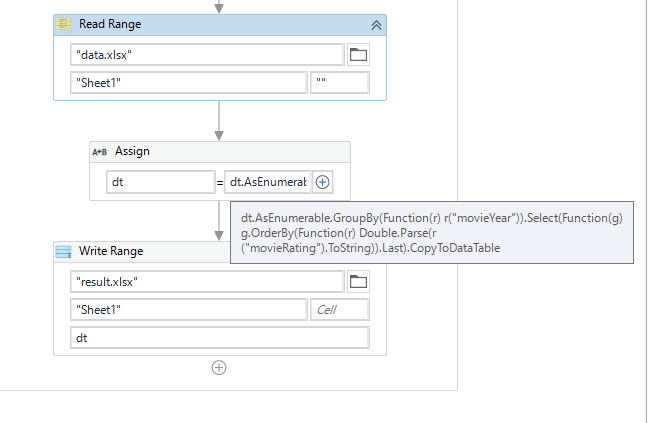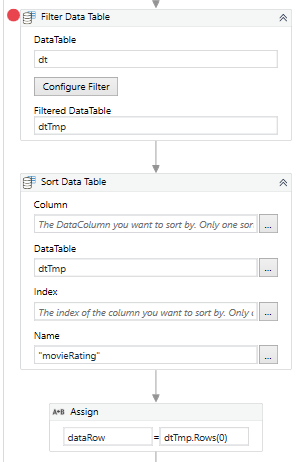# Compare two columns with same values

Good morning Uipathers!

my bot, in the end of the process, needs to send an e-mail with the best rated movie of each year, happens that in the column “movieYear” we get a lot of same years. I was trying to use filterDataTable but just realize I don’t how to do the logic there and also couldt find a query to do it. This is the column, how would you do it?thank you!try this
Main (1).xaml (11.6 KB)
logic:

1. create new datatable dtNew= dt.Clone
2. loop over unique years of dt, for each year find row with max rating and add to dtNew

Hi,

Can you try the following expression?``````dt = dt.AsEnumerable.GroupBy(Function(r) r("movieYear")).Select(Function(g) g.OrderBy(Function(r) Double.Parse(r("movieRating").ToString)).Last).CopyToDataTable
``````

Regards,

could you explain that query pls?this version is by using filter datatable + sort datatable
(same logic as above)
just that i replaced

`dataRow = dt.AsEnumerable.Where(function(x) x("movieYear").ToString.equals(year)).OrderByDescending(function(y) CDbl(y("movieRating"))).CopyToDataTable.Rows(0)`

withMain (1).xaml (13.5 KB)

1 Like

Hi,

could you explain that query pls?

`````` dt.AsEnumerable.GroupBy(Function(r) r("movieYear"))
``````

The above returns Grouping by movie year. For example, from the above sample, key of the group is 2001 and its content is rows of “in a coma…” and “A look at …”. Other group which has key such as 2004,2007 etc are same.

``````Select(Function(g) g.OrderBy(Function(r) Double.Parse(r("movieRating").ToString)).Last)
``````

this processes to sort ascend by movieRating and returns last row to each group. It means row of the highest rating will be returned in each group.

Finally, we can get datatable of the highest rating in each year.

Regards,

1 Like

brilliant! thank you a lot! ありがとう ございます1 Like

Hey, Sorry to bother! I kept trying the the expression but it keeps giving same error:

Hi,

I guess your data contains non-numeric value(include blank). For now, can you try the following expression?

``````dt.AsEnumerable.GroupBy(Function(r) r("movieYear")).Select(Function(g) g.OrderBy(Function(r) if( Double.TryParse(r("movieRating").ToString,New Double),Double.Parse(r("movieRating").ToString),0)).Last).CopyToDataTable
``````

Regards,

1 Like

worked perfectly! many thanks, for real! If you may let me: why new Double?This topic was automatically closed 3 days after the last reply. New replies are no longer allowed.